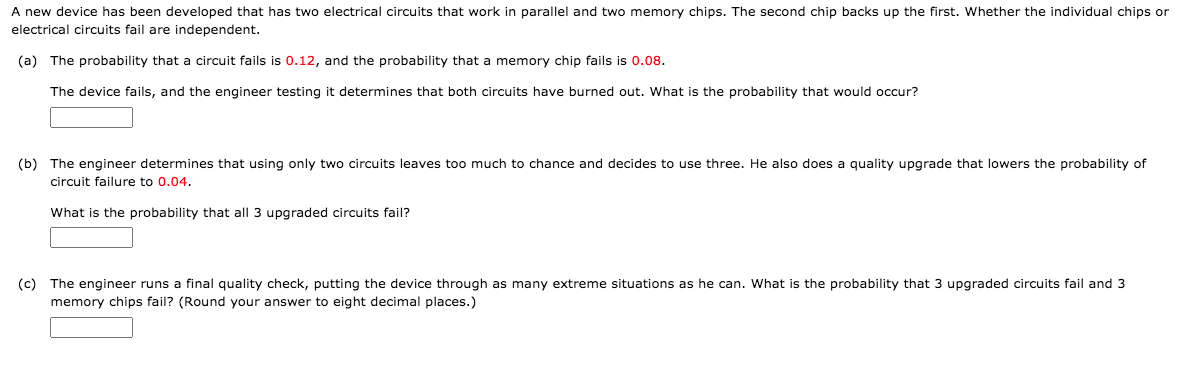# A new device has been developed that has two electrical circuits that work in parallel and two memory chips. The second chip backs up the first. Whether the individual chips or electrical circuits fail are independent. (a) The probability that a circuit fails is 0.12, and the probability that a memory chip fails is 0.08. The device fails, and the engineer testing it determines that both circuits have burned out. What is the probability that would occur? (b) The engineer determines that using only two circuits leaves too much to chance and decides to use three. He also does quality upgrade that lowers the probability of circuit failure to 0.04. What is the probability that all 3 upgraded circuits fail? (c) The engineer runs a final quality check, putting the device through as many extreme situations as he can. What is the probability that 3 upgraded circuits fail and 3 memory chips fail? (Round your answer to eight decimal places.)

Questionhelp_outlineImage TranscriptioncloseA new device has been developed that has two electrical circuits that work in parallel and two memory chips. The second chip backs up the first. Whether the individual chips or electrical circuits fail are independent. (a) The probability that a circuit fails is 0.12, and the probability that a memory chip fails is 0.08. The device fails, and the engineer testing it determines that both circuits have burned out. What is the probability that would occur? (b) The engineer determines that using only two circuits leaves too much to chance and decides to use three. He also does quality upgrade that lowers the probability of circuit failure to 0.04. What is the probability that all 3 upgraded circuits fail? (c) The engineer runs a final quality check, putting the device through as many extreme situations as he can. What is the probability that 3 upgraded circuits fail and 3 memory chips fail? (Round your answer to eight decimal places.) fullscreen

### Want to see this answer and more?

Experts are waiting 24/7 to provide step-by-step solutions in as fast as 30 minutes!*

*Response times may vary by subject and question complexity. Median response time is 34 minutes for paid subscribers and may be longer for promotional offers.
Tagged in
Math
Statistics

### Other